Next: 4.2.3 Zernike Polynomials for Up: 4.2 Lens Aberrations and Previous: 4.2.1 Defocus

## 4.2.2 Power Series Representation of Primary Seidel Aberrations

The common way to describe the five primary or Seidel aberrations is a power series expansion of the aberration function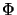(n, m) [11, pp. 211-218]. It is convenient to scale the incident wavevector inm in the image space with the numerical aperture NA of the imaging lens first, i.e.,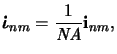(4.63)

which has the advantage that the pass-region of the pupil function (cf. (4.53) and (4.58)) transforms in the scaled domain into the unit circle: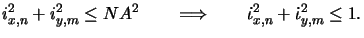(4.64)

Hence, the optical path difference of the most obliquely incident ray does not depend on the numerical aperture and the resulting formulae provide clear information on the impact of each aberration type.

The power series expansion of the aberration function takes the form [117,118]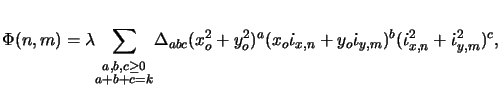(4.65)

whereby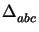denotes the maximal optical path difference in units of the wavelength between the rays entering in the center of the lens and at the boundary, while (xo, yo) is the position of the mask with respect to the optical axis. In the power series only polynomials of degree 2k in the three variables ro =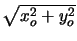,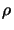=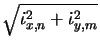, and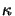=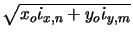occur. A term of a particular degree 2k is said to represent a wave aberration of order 2k. The aberrations of lowest order (2k = 4) are usually called primary or Seidel aberrations. As can be seen from (4.74) five terms with a + b + c = 2 exist. In Table 4.1 a summary of the Seidel aberrations including the resulting image shift is described.

Table 4.1: Seidel aberrations are described by power series expansion of the aberration function(n, m). The position of best focus is shifted by (Rfcos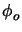, Rfsin, Zf).

 Focal shift Aberration (a, b, c)(n, m) Rf Zf Spherical (0,0,2)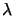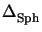(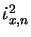+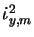)2 0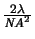Coma (0,1,1)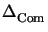(xo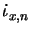+ yo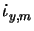)(+)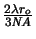0 Astigmatism (0,2,0)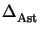(xo+ yo)2 0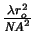Curvature(1,0,1)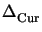(xo2 + yo2)(+) 0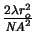Distortion (1,1,0)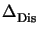(xo2 + yo2)(xo+ yo)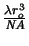0For a fixed object position curvature equals approximate'' defocus (cf. (4.71)), whereby the amount of defocus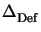is related to the optical path differenceby= 2ro2/NA2.

Although the expansion of (4.74) is a general representation of the aberration function in that any aberration function(n, m) can be represented, other polynomials are more suited to describe higher-order aberration terms.Next: 4.2.3 Zernike Polynomials for Up: 4.2 Lens Aberrations and Previous: 4.2.1 Defocus
Heinrich Kirchauer, Institute for Microelectronics, TU Vienna
1998-04-17